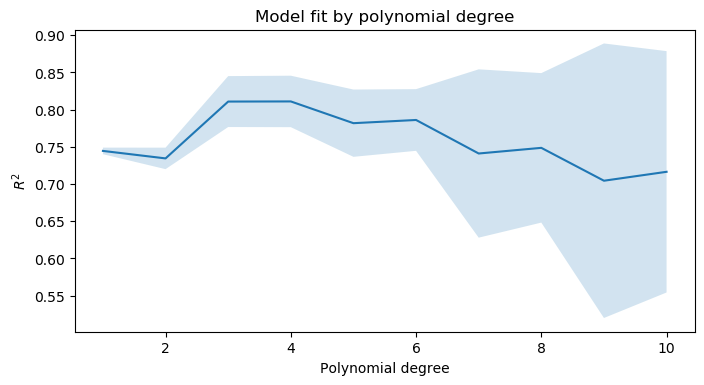# scikit-learn

``````import numpy as np
import pandas as pd
import matplotlib.pyplot as plt

from sklearn.preprocessing import PowerTransformer
from sklearn.compose import ColumnTransformer
from sklearn.pipeline import Pipeline
from sklearn.linear_model import LinearRegression, Ridge, RidgeCV
from sklearn.model_selection import GridSearchCV, KFold
``````

## General

### Train–Test split

``````from sklearn.model_selection import train_test_split
from sklearn.datasets import make_regression

X, y = make_regression(n_features=1)
X_train, X_test, y_train, y_test = train_test_split(X, y, test_size=0.20)
``````

## Feature engineering

### Apply scikit-learn preprocessing with keeping index and columns of DataFrame

Scikit-learn includes a bunch of useful feature transformation functions such as `PolynomialFeatures` and `OneHotEncoder`. They are easy to use as part of a model pipeline, but their intermediate outputs (numpy matrices) can be difficult to interpret. I find havng these intermediate outputs back in a pandas DataFrame with the original index and column names is helpful when troubleshooting or communicating the results of preprocessing.

``````from sklearn.preprocessing import PolynomialFeatures, FunctionTransformer

df = pd.DataFrame({'category': ['a', 'b', 'c'], 'numeric': np.random.random(size=3)})
display(df)

def pandas_polynomial_features(X):
""" Wrapper for calling PolynomialFeatures while preserving
index and column names from input DataFrame. """
poly_tx = PolynomialFeatures(degree=2, include_bias=False).fit(X)
poly_cols = poly_tx.get_feature_names(input_features=X.columns)
return pd.DataFrame(poly_tx.transform(X), index=X.index, columns=poly_cols)

PandasPolynomialFeatures = FunctionTransformer(pandas_polynomial_features, validate=False)

numeric_cols = df.select_dtypes(np.number).dtypes.index.values.tolist()
PandasPolynomialFeatures.fit_transform(df[numeric_cols])
``````

category numeric
0 a 0.843190
1 b 0.169204
2 c 0.222390

numeric numeric^2
0 0.843190 0.710969
1 0.169204 0.028630
2 0.222390 0.049457

### Getting readable metadata from `OneHotEncoder`

Fitted `OneHotEncoder` objects do have a `categories_` attribute, but it only includes the “child” value without reference to the “parent” categorical variable. If you are working with categoricals whose values overlap (e.g. departure and arrival city of a trip) this can become confusing. I prefer the column names to include the initials of the initial column separated by `_is_`, to aid in interpretability during later phases.

``````df = pd.DataFrame({'home_city': np.random.choice(['toronto', 'paris', 'london'], size=5),
'fav_city': np.random.choice(['toronto', 'paris', 'london'], size=5)}, dtype='category')

display(df)
``````

home_city fav_city
0 london paris
1 toronto paris
2 paris london
3 paris paris
4 toronto paris
``````from sklearn.preprocessing import OneHotEncoder

def pandas_one_hot_encoder(X):

""" Wrapper for calling PolynomialFeatures while preserving index and column names from input DataFrame. """

fit_obj = OneHotEncoder().fit(X)
nested_output_col_names = fit_obj.categories_
flat_output_col_names = []

for cat_col, corresp_enc_cols in zip(X.columns, nested_output_col_names):
stem = ''.join([x for x in cat_col.split('_')]) + '_is_'
flat_output_col_names.extend(stem + corresp_enc_cols)

return pd.DataFrame(fit_obj.transform(X).toarray(),
index=X.index,
columns=flat_output_col_names)

PandasOneHotEncoder = FunctionTransformer(pandas_one_hot_encoder, validate=False)
``````

hc_is_london hc_is_paris hc_is_toronto fc_is_london fc_is_paris
0 1.0 0.0 0.0 0.0 1.0
1 0.0 0.0 1.0 0.0 1.0
2 0.0 1.0 0.0 1.0 0.0
3 0.0 1.0 0.0 0.0 1.0
4 0.0 0.0 1.0 0.0 1.0

### Using ColumnTransformer from inside a pipeline

Scikit-learn has recently introduced `ColumnTransformer` , which is a very useful tool for performing preprocessing on a dataset with heterogeneous dtypes. Previously this was cumbersome without using something like sklearn-pandas. It is not yet perfectly integrated with the rest of the sklearn API though. Recently I was trying to use `ColumnTransformer` as part of a `Pipeline` object, but encountered the following error:

``````ValueError: Specifying the columns using strings is only supported for pandas DataFrames
``````

The `transformers` parameter of `ColumnTransformer` takes a list of three-element tuples, the third of which points towards which columns should be passed into each respective step. When you pass a pandas DataFrame directly to the transformer via its `.fit()` method, you can use a list of column names as this third element in each tuple. But if you are using `ColumnTransformer` as part of a pipeline, these have to be numeric indices.

``````df = pd.DataFrame({'home_city': np.random.choice(['toronto', 'paris', 'london'], size=5),
'fav_city': np.random.choice(['toronto', 'paris', 'london'], size=5)}, dtype='category')

df['age'] = np.random.randint(80, size=5)
display(df)

numeric_cols = df.dtypes.apply(lambda x: x.kind in 'bifc').reset_index(drop=True).loc[lambda x: x == True].index
cat_cols = (df.dtypes == 'category').reset_index(drop=True).loc[lambda x: x == True].index

col_tx = ColumnTransformer(transformers=[
('num', PowerTransformer(), numeric_cols),
('cat', OneHotEncoder(drop='first', handle_unknown='error'), cat_cols)
])

pipe = Pipeline([
('col_tx', col_tx),
('model', LinearRegression())
])

pipe.fit(df, [0, 1, 0, 1, 0]);
``````

home_city fav_city age
0 toronto paris 58
1 toronto toronto 23
2 london toronto 68
3 paris london 75
4 paris paris 7

### Parameter search on pre-processing steps

It is straightforward to use `GridSearchCV` on final model parameters, but it is also possible to use it for parameters of “upstream” data transformations, if you compose them together with the final model into a `Pipeline` object. Then you can use use the syntax `<estimator>__<param>` separated by a double underscore in your `param_dict` keys to specify which parameter belongs to which step of the pipeline. Read more at the official scikit-learn docs.

``````from sklearn.datasets import make_regression

def find_best_degrees(X, y, degrees=range(1, 11)):
""" Example of grid search on parameters of an upstream transformation step in a pipeline. """

pipe = Pipeline([
('poly_tx', PolynomialFeatures(include_bias=False)),
('model', Ridge(normalize=True))
])

param_grid = {'poly_tx__degree': degrees}
search = GridSearchCV(pipe, param_grid, cv=KFold(n_splits=5, shuffle=True)).fit(X, y)

avg_scores = search.cv_results_['mean_test_score']
std_scores = search.cv_results_['std_test_score']

fig, ax = plt.subplots(figsize=(8, 4), dpi=100)
ax.plot(degrees, avg_scores, label='\$R^2\$')
ax.fill_between(degrees, avg_scores-std_scores, avg_scores+std_scores, alpha=0.2)
ax.set_title('Model fit by polynomial degree')
ax.set_ylabel('\$R^2\$')
ax.set_xlabel('Polynomial degree')

X, y = make_regression(n_features=1)
find_best_degrees(X, y)
``````## Package versions

This notebook was run with the following versions.

``````%reload_ext watermark
%watermark -v -p numpy,pandas,sklearn,matplotlib
``````
``````CPython 3.7.7
IPython 7.13.0

numpy 1.18.1
pandas 1.0.3
sklearn 0.22.1
matplotlib 3.1.3
``````